# Class 8 RD Sharma Solutions – Chapter 3 Squares and Square Roots – Exercise 3.3 | Set 2

### (i) 425

Solution:

425 = 420 + 5 = (42 x 10) + 5

Here, n = 42

=> n(n + 1) = 42(42 + 1) = 42 x 43 = 1806

=> 4252 = 180625

### (ii) 575

Solution:

575 = 570 + 5 = (57 x 10) + 5

Here, n = 57

=> n(n + 1) = 57(57 + 1) = 57 x 58 = 3306

=> 5752 = 330625

### (iii) 405

Solution:

405 = 400 + 5 = (40 x 10) + 5

Here, n = 40

=> n(n + 1) = 40(40 + 1) = 1640

=> 4052 = 164025

### (iv) 205

Solution:

205 = 200 + 5 = (20 x 10) + 5

Here, n = 20

=> n(n + 1) = 20(20 + 1) = 420

=> 2052 = 42025

### (v) 95

Solution:

95 = 90 + 5 = (9 x 10) + 5

Here, n = 9

=> n(n + 1) = 9(9 + 1) = 90

=> 952 = 9025

### (vi) 745

Solution:

745 = 740 + 5 = (74 x 10) + 5

Here, n = 74

=> n(n + 1) = 74(74 + 1) = 5550

=> 7452 = 555025

### (vii) 512

Solution:

512² = (250 + 12)1000 + (12)²

= (262)1000 + 144

= 262000 + 144

= 262144

Hence, 5122 = 262144

### (viii) 995

Solution:

995 = 990 + 5 = (99 x 10) + 5

Here, n = 99

=>n(n + 1) = 99(99 + 1) = 9900

9952 = 990025

### (i) 405

Solution:

405 = 400 + 5

Here, a = 400, b = 5

Using the identity, (a + b)2 = a2 + 2ab + b2

4052 = 4002 + (2 x 400 x 5) + 52

= 160000 + 4000 + 25

= 164025

Thus, 4052 = 164025

### (ii) 510

Solution:

510 = 500 + 10

Here, a = 500, b = 10

Using the identity, (a + b)2 = a2 + 2ab + b2

5102 = 5002 + (2 x 500 x 10) + 102

= 250000 + 10000 + 100

= 260100

Thus, 5102 = 260100

### (iii) 1001

Solution:

1001 = 1000 + 1

Here, a = 1000, b = 1

Using the identity, (a + b)2 = a2 + 2ab + b2

10012 = 10002 + (2 x 1000 x 1) + 12

= 1000000 + 2000 + 1

= 1002001

Thus, 10012 = 1002001

### (iv) 209

Solution:

209 = 200 + 9

Here, a = 200, b = 9

Using the identity, (a + b)2 = a2 + 2ab + b2

2092 = 2002 + (2 x 200 x 9) + 92

= 40000 + 3600 + 81

= 43681

Thus, 2092 = 43681

### (v) 605

Solution:

605 = 600 + 5

Here, a = 600, b = 5

Using the identity, (a + b)2 = a2 + 2ab + b2

6052 = 6002 + (2 x 600 x 5) + 52

= 360000 + 6000 + 25

= 366025

Thus, 6052 = 366025

### (i) 395

Solution:

395 = 400 – 5

Here, a = 400, b = 5

Using the identity, (a – b)2 = a2 – 2ab + b2

3952 = (400 – 5)2 = 4002 – (2 x 400 x 5) + 52

= 160000 – 4000 + 25

= 156025

Thus, 3952 = 156025

### (ii) 995

Solution:

995 = 1000 – 5

Here, a = 1000, b = 5

Using the identity, (a – b)2 = a2 – 2ab + b2

9952 = (1000 – 5)2 = 10002 – (2 x 1000 x 5) + 52

= 1000000 – 10000 + 25

= 990025

Thus, 9952 = 990025

### (iii) 495

Solution:

495 = 500 – 5

Here, a = 500, b = 5

Using the identity, (a – b)2 = a2 – 2ab + b2

4952 = (500 – 5)2 = 5002 – (2 x 500 x 5) + 52

= 250000 – 5000 + 25

= 245025

Thus, 4952 = 245025

### (iv) 498

Solution:

498 = 500 – 2

Here, a = 500, b = 2

Using the identity, (a – b)2 = a2 – 2ab + b2

4982 = (500 – 2)2 = 5002 – (2 x 500 x 2) + 22

= 250000 – 2000 + 4

= 248004

Thus, 4982 = 248004

### (v) 99

Solution:

99 = 100 – 1

Here, a = 100, b = 1

992 = (100 – 1)2 = 1002 – (2 x 100 x 1) + 12

= 10000 – 200 + 1

= 9801

Using the identity, (a – b)2 = a2 – 2ab + b2

Thus, 992 = 9801

### (vi) 999

Solution:

999 = 1000 – 1

Here, a = 1000, b = 1

Using the identity, (a – b)2 = a2 – 2ab + b2

9992 = (1000 – 1)2 = 10002 – (2 x 1000 x 1) + 12

= 1000000 – 2000 + 1

= 998001

Thus, 9992 = 998001

### (vii) 599

Solution:

599 = 600 – 1

Here, a = 600, b = 1

Using the identity, (a – b)2 = a2 – 2ab + b2

5992 = (600 – 1)2 = 6002 – (2 x 600 x 1) + 12

= 360000 – 1200 + 1

= 358801

Thus, 5992 = 358801

### (i) 52

Solution:

52 = 50 + 2

Here, a = 50, b = 2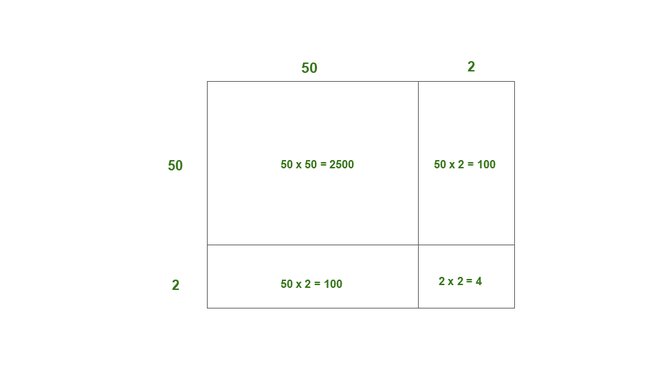Thus, the area of square of side 52 = 2500 + 100 + 100 + 4

= 2704

Thus, 522 = 2704

### (ii) 95

Solution:

95 = 90 + 5

Here, a = 90, b = 5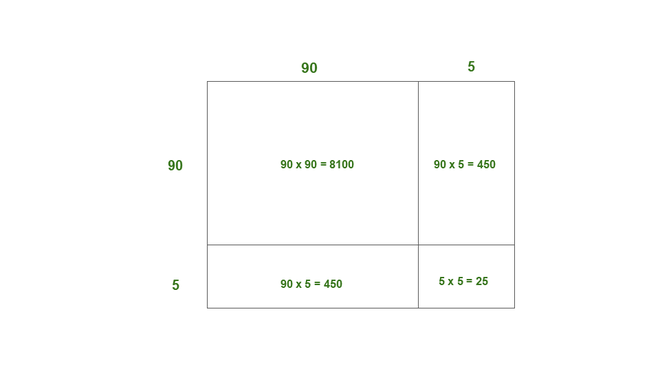Thus, the area of square of side of 95 = 8100 + 450 + 450 + 25

= 9025

Thus, 952 = 9025

### (iii) 505

Solution:

505 = 500 + 5

Here, a = 500, b = 5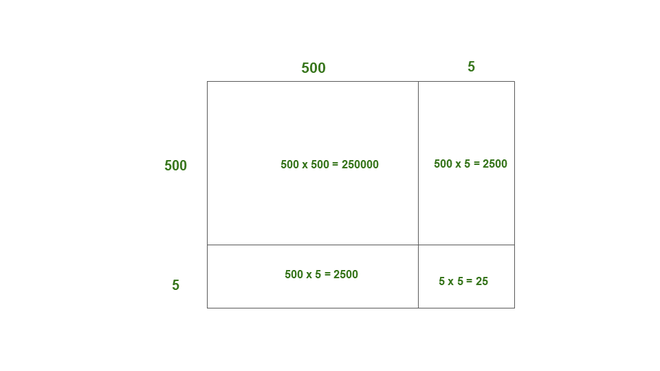Thus, the area of square of side 505 = 250000 + 2500 + 2500 + 25

= 255025

Thus, 5052 = 255025

### (iv) 702

Solution:

702 = 700 + 2

Here, a = 700, b = 2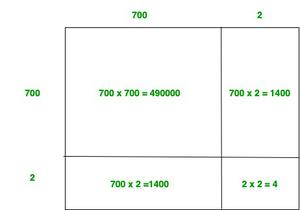Thus, the area of square of side 702 = 490000 + 1400 + 1400 + 4

= 492804

Thus, 7022 = 492804

### (v) 99

Solution:

99 = 90 + 9

Here, a = 90, b = 9.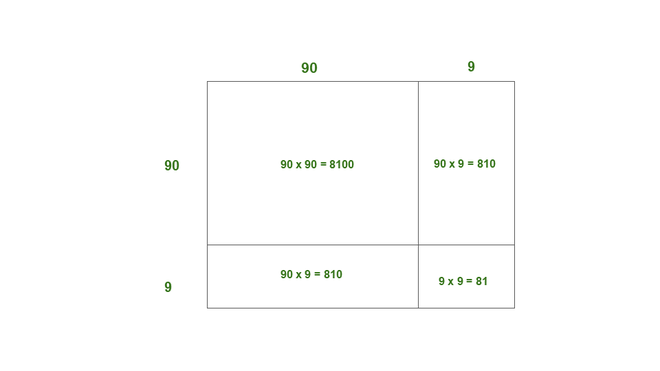Thus, the area of square of side of 99 = 8100 + 810 + 810 + 81

= 9801

Thus, 992 = 9801

Whether you're preparing for your first job interview or aiming to upskill in this ever-evolving tech landscape, GeeksforGeeks Courses are your key to success. We provide top-quality content at affordable prices, all geared towards accelerating your growth in a time-bound manner. Join the millions we've already empowered, and we're here to do the same for you. Don't miss out - check it out now!

Previous
Next